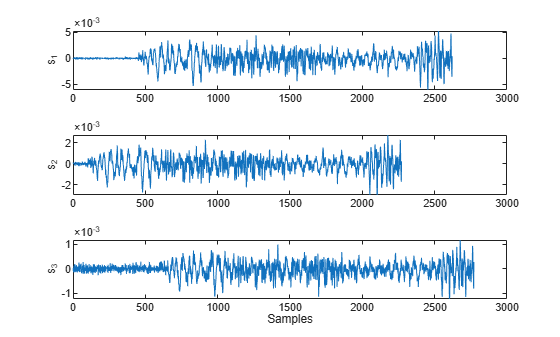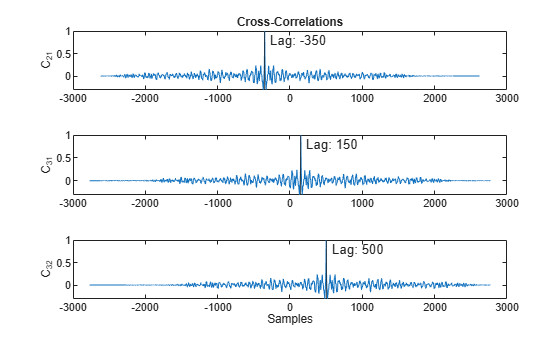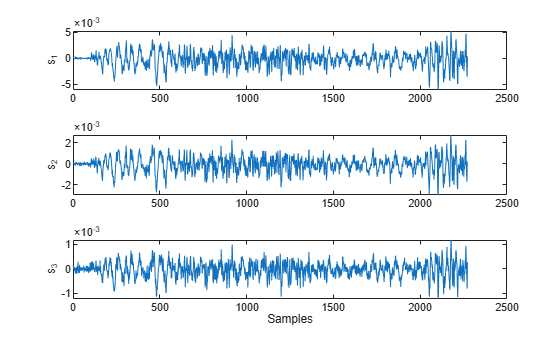Align Signals Using Cross-Correlation

Many measurements involve data collected asynchronously by multiple sensors. If you want to integrate the signals and study them in tandem, you have to synchronize them. Use xcorr for that purpose.

For example, consider a car crossing a bridge. The vibrations it produces are measured by three identical sensors located at different spots. The signals have different arrival times.

Load the signals into the MATLAB® workspace and plot them.

ax(1) = subplot(3,1,1);
plot(s1)
ylabel('s_1')
axis tight

ax(2) = subplot(3,1,2);
plot(s2)
ylabel('s_2')
axis tight

ax(3) = subplot(3,1,3);
plot(s3)
ylabel('s_3')
axis tight
xlabel('Samples')Compute the cross-correlations between the three pairs of signals. Normalize them so their maximum value is 1.

[C21,lag21] = xcorr(s2,s1);
C21 = C21/max(C21);

[C31,lag31] = xcorr(s3,s1);
C31 = C31/max(C31);

[C32,lag32] = xcorr(s3,s2);
C32 = C32/max(C32);

The locations of the maximum values of the cross-correlations indicate time leads or lags.

[M21,I21] = max(C21);
t21 = lag21(I21);

[M31,I31] = max(C31);
t31 = lag31(I31);

[M32,I32] = max(C32);
t32 = lag31(I32);

Plot the cross-correlations. In each plot display the location of the maximum.

subplot(3,1,1)
plot(lag21,C21,[t21 t21],[-0.5 1],'r:')
text(t21+100,0.5,['Lag: ' int2str(t21)])
ylabel('C_{21}')
axis tight
title('Cross-Correlations')

subplot(3,1,2)
plot(lag31,C31,[t31 t31],[-0.5 1],'r:')
text(t31+100,0.5,['Lag: ' int2str(t31)])
ylabel('C_{31}')
axis tight

subplot(3,1,3)
plot(lag32,C32,[t32 t32],[-0.5 1],'r:')
text(t32+100,0.5,['Lag: ' int2str(t32)])
ylabel('C_{32}')
axis tight
xlabel('Samples')s2 leads s1 by 350 samples; s3 lags s1 by 150 samples. Thus s2 leads s3 by 500 samples. Line up the signals by clipping the vectors with longer delays.

s1 = s1(-t21:end);
s3 = s3(t32:end);

ax(1) = subplot(3,1,1);
plot(s1)
ylabel('s_1')
axis tight

ax(2) = subplot(3,1,2);
plot(s2)
ylabel('s_2')
axis tight

ax(3) = subplot(3,1,3);
plot(s3)
ylabel('s_3')
axis tight
xlabel('Samples')